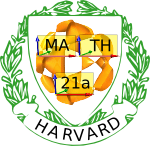Fall 2005

# Mathematics Math21a Fall 2005

## Multivariable Calculus

Office: SciCtr 434
Email: knill@math.harvard.edu
Harvard Mathematics

 Weekly checklists: "Somewhere, over the rainbow ..."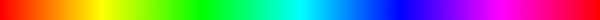Week1 Week2 Week3 Week4 Week5 Week6 Week7 Week8 Week9 Week10 Week9 Week10

 Send questions of potential general interest to math21a@fas.harvard.edu.
 Question: Q: What is the curve?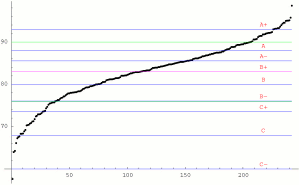Answer: This is the MFAQ, the most frequently asked question. As you know, Harvard, as many other colleges, experiences grade inflation. This is unfortunate, because it allows students less and less to gauge their progress and for students near the AB treshold, it is essentially a matter of luck on which side they fall. The quantisation by letter grades is too rough and with time, most of the grades had been pushed into the A-B range. This discourages top students to work harder (I already have an A) and students with a B grade (I can not get into the A grade). A simple solution to the problem would be to get rid of the quantisation and to submit a number between 0-100 to the registrar, forcing the distribution to have a fixed mean and standard deviation. But the world is not perfect and so, every teacher at the end of the semester ponders over the problem to figure out a "curve". I keep three constraints fixed: there should be maximally 40 percent A and A- and the average should be near the threshold of B to B+. Furthermore, the standard deviation window should include only A and B grades. Then, according to the distribution, the cutoffs are set. This is usually not fixed from year to year. It depends on how difficult the exams are percieved, where natural flat parts of the curve are and depends at the analysis of grades of a few "gauge" students, I look at closely and where I can see what was the reason for success or for difficulties.
 Question: Q: Why not fix and publish the cutoffs of the curve beforehand? Answer: In other words, why not skip the "curve" at all? It would be possible but a bit risky, especially with a resurrection policy. It is very reasonable in courses, where the outcome of exams can be precicely predicted (courses with a lot of memorizing material or routine problems like in early calculus courses), but not in courses, where the material is more complex and the exams are more conceptual. It can be even less predicted when projects are included. In multivariable calculus, it is more difficult to write exams with a predicted outcome than in a course like 1a. I aim for a 75 average score. For an over 80 percent average, the exam was too easy or too close to practice exams or exams from the previous years (which are assumed to be known) for an average below 70 percent, the exam was too hard, confusing or there were time constraints during the exam. Since our HW scores usually high, we end up with an over 80-85% average final score. In this course, we had quite a typical distribution, but due to the TF problems, it had been very hard to get close to 100%. The multple choice parts make it also difficult to have a really low score.
Question: Q: Why is this course so competitive? Answer: Some degree of competition is a good motivator. Most Harvard students are competitive. Otherwise, they would not be here. But too much competition can be tough too. If you feel stressed, use your teacher more as a coach during the semester. He or she can tell you how to learn faster or how to take short cuts. As in sports, a good coach can help.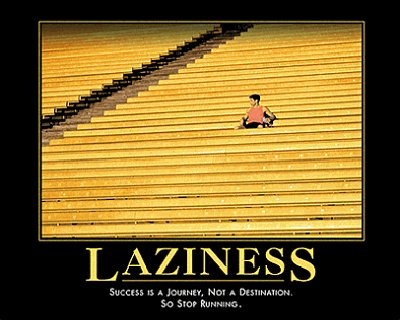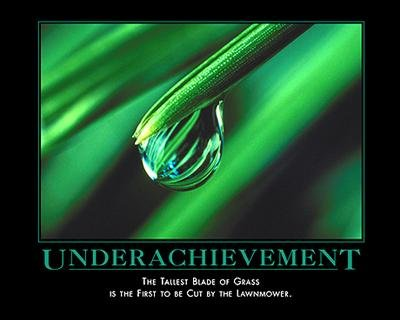Question: Q: Do you have statistics about resurrection? Answer: Sure, we have statistics about everything. Resurrection happened rarely. This is clear because the HW score is in general high so that beating the average grade with the final grade was not easy. But rusurrection happend. It could happen when hard work with the tougher material of the third part could make a difference. The graphics to the left shows the correlation between average score and final score. The graphics to the left shows the difference of the final score minus the average score.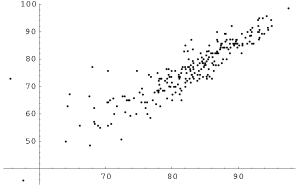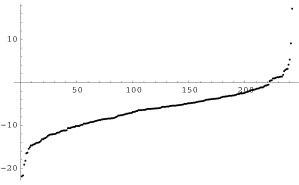Question: Q: How much line integrals do the Biochem sections have to know? Answer: Everything inlcluding the fundamental theorem of line integrals that is until and including section 13.2. Greens theorem (section 13.4) is no more part of the final exam for Biochem sections. The fundamental theorem of line integrals 13.3 might not have been mentioned also in some sections. So, 13.3 is also not part of the exam.
 Question: Q: Will the review sessions be taped? Answer: We try. It is never clear however whether the videos can be put online on time. Meddia services are running on a smaller staff than usual during reading period because many students working there have also exams. So, please try to come or assign somebody to take notes for you.
 Question: Q: Do TTh sections also have three jokers? Answer: Yes, this needs to be clarified: to be fair, both MWF and TTh sections have 1 week worth of their lowest HW scores as "jokers". So, three hours for MWF correponds most weeks to 2 HW for TuThu. We take the same percentage off from the bottom scores from everybody and this percentage is measured by 3 hours for MWF.
 Question: Q: Are the midterms curved? Answer: No. The final grade will be curved according to the class distribution. You find the formulas on the website and you can do the math yourself. Most of the time in the past, a final grade of 90 or more guarantees a A grade. This could theoretically change if the final exam would have a 95 average but you can bet that the final exam will have a similar difficulty than the midterms and accordingly a similar mean.
 Question: Q: I'm trying to graph an implicit surface in Mathematica, but although I do everything as it is in the notebook, it does not work. Answer: This is a common problem. The implicit plot requires you to load a library first. If you try first to run the command without the library, it will no more load the library. Save your notebook and start it again but make sure that you run the command which imports the library before. Here is a simple example: start a new notebook and enter the following two commands: ```Get["Graphics`ContourPlot3D`"]; ContourPlot3D[x^2+y^2+z^2-4,{x,-3,3},{y,-3,3},{z,-3,3}] ``` It will give you an implicit plot.
 Question: Q: Which sections in the book are skipped in the book for this course? Answer: You can correlate the syllabus with the book index. But it is easier to tell, what we do not cover: Keplers laws in 10.4. General change of coordinates (section 12.9) In section 13.2, we do not look at line integrals of the form int f ds, where f is a scalar function. These are generalized length integrals and a million times less relevant than the actual line integrals. Line integrals for us always involve a vector field F and are of the form int F(r(t) r'(t) dt. You can safely ignore any line integral of the form int f ds. Believe us, the subject is difficult enough without them. In section 13.6, we do not look at surface integrals of the form int f |r_u x r_v| du dv These are generalized surface area integrals and a million times less relevant than the actual surface integrals int F(r(u,v)) (r_u x r_v) du dv. you can safely ignore any surface integral which involves scalar functions f. Believe us, the subject is difficult enough without them.
 Question: Q: Is it true that the material in the biochem section is much easier than the material in the regular and physics sections during the last two weeks? Answer: We try to have all sections to do the same amount of work and keep things on a similar difficulty level. Because the subjects are very different, this is not easy. In the past, both cases occured. There were years, where the biochem section material (a basic introduction to probability theory) was easier. In other years it had been harder. Because part of the final exam will be split. That is: 2-3 questions will be different for the biochem sections than for the other sections, the fairness of the exam is an important issue. In order that nobody has to worry about "being in the wrong section" or having had an unfair final exam", the final exam is split into two parts, one common part and one which is flavour dependent. Subtotals are computed for the common exam as well as subtotals for the part which is split. The second part will be curved seperately in such a way that the average of the biochem class and the regular class are the same.
 Question: Q: What does this Stallone movie have to do with Math? Answer: First of all, the movie made it to the Golden Raspberry Awards. THen, climbing has a lot to do with gradients. The fastest way to the top is going in the direction of the gradient, if z=f(x,y) is the height function. If you fall, that happens along the "steepest decent". Note that the mountain in the movie is a surface which is not a graph of a function. In order to describe it, you would have to write it as a level surface g(x,y,z)=0, or parametrize it.
 Question: Q: How much should I read about the chain rule? Answer: As little as possible! Just keep the one dimensional chain rule d/dt f(g(t)) = f'(g(t)) g'(t) in mind. The only new chain rule, we ever need in this course is d/dt f(r(t)) = nabla f(r(t)) . r'(t) It is the usual chain rule, where f' is replaced by the gradient of f and r'(t) is the velocity of the curve r(t). It is an interesting phenonmenon, that if students start learning about the multivariable chain rule, they lose even the ability to do the one dimensional chain rule. Chaos takes over in the brains, the end of the world is near etc. etc. And all because of that wicked chapter 11.5. Dont read it!Question: Q: Why is the second midterm also in the same time as the life science exam? Answer: Cruelty of course! No, seriously, when we start organizing a large course like 21a, the first thing we do is to organize the rooms, at least three months before the actual exam. We did that early August for this course. All the rest of the organization of 21a, the syllabus etc depend crucially on these dates. There are not so many days eligible for midterm exam dates due to a few religious holidays and due to syllabus constraints. But the main issue is finding an exam room for 250-300 students. The life science exam happens during a regular class and it would room-wise have been possible to move those dates because the rooms are anyway booked for classes. But changing an announced exam date is always problematic, after the semester has started, hundreds of people have organized their calendars already. We would not have changed the exam dates even in September, since sport coaches or art directors might already have had a look at the exam schedules. To prepair for a day with two exams, we advise start early with both exam preparations. By the way, during final exams, you will most likely have two exams at the same day. Take the opportunity to fine tune your time management skills now.
 Question: Q: What do we do if we have to compute the directional derivative D_u f when u is not a unit vector? Answer: The book defines the directional derivative only for unit vectors. We extend this definition for all vectors u, so that the formula D_u f = nabla f . u holds for all vectors u. The reason why calculus books define directional derivatives for unit vectors only is that unit vectors provide a "direction". The reason for extending the definition is not only mathematical elegance but also that with a narrow definition, students always want to divide by the length of the vector.
 Question: Q: How much do I have to know about PDE's (Partial differential equations) Answer: The website has two one page handouts on PDE's. Read them. You should know, what a PDE is, especially be able to distinguish a PDE with an ODE (ordinary differential equations). You should also know a few PDE's like the heat equation u_t = u_xx , wave equation u_tt = u_xx, advection equation u_t = u_x, Burber equation u_t + u u_x = u_xx, laplace equation u_xx + u_yy=0, Schroedinger equation u_t = i h u_xx + V(x). You should be able to verify that a specific function is a solution to a given PDE. Finally, you should also have an idea what these PDEs describe. You have not to know in this course, how we find solutions to PDE's. The next math course Math21b will teach you that for the above PDE's.
 Question: Q: I have trouble graphing surfaces, especially parametrized surfaces. Answer: Graphing surfaces by hand is difficult. But can be fun and challenging to draw a good picture of a surface. Traces can help you to visualize the surface. Use Mathematica for more complicated surfaces. Here is a list of surfaces, you should be able to draw by hand: plane, all quadrics, surfaces of revolution, the torus (doughnut), graphs of simple functions f(x,y).
 Question: Q: I did not get the book yet. What should I do? Answer: The Coop had temporarily not enough books on hold. Contact math21a@fas.harvard.edu, so that we can get you the first weeks homework set in a different form.
 Question: Q: Can I take the course Pass/Fail? Answer: While this was ruled out at the organisatorial meeting, it can be an option in very exceptional cases. Please contact the course head for advise in this matter.
 Question: Q: Can I use the old edition of the book? Answer: We need the third edition. The problems are different from edition to edition.
 Question: Q: Do biochem sections need a second book? Answer: Technically, it is optional. But since it costs 9+ dollars only, why not get it?
 Please send comments to math21a@fas.harvard.edu Background music: Pictures at an Exhibition" by the Russian composer Modest Petrovich Mussorgsky.
 Math21a, Multivariable Calculus, Fall 2005, Department of Mathematics, Faculty of Art and Sciences, Harvard University

Sat Jan 21 22:11:28 EST 2006